RANDOM Statement

RANDOM random-effectdistribution SUBJECT=variable <options> ;

The RANDOM statement defines a single random effect and its prior distribution or an array of random effects and their prior distribution. The random-effect must be represented by either a symbol or an array that appears in your SAS programming statements. The RANDOM statement must consist of a symbol for a random effect (or an array for multivariate random effects), a tilde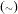, the distribution for the random effect, and then a SUBJECT= variable.

You can specify multiple RANDOM statements. Not all distributions supported in the MODEL statement are avaliable for the RANDOM statement. Table 54.4 shows the valid distributions.

Table 54.4 Valid Distributions in the RANDOM Statement

Distribution Name

Definition

beta(<a=>, <b=>)

Beta distribution with shape parametersandbinary(<prob|p=>)

Binary (Bernoulli) distribution with probability of success. You can use the alias bern for this distribution.

gamma(<shape|sp=> a, scale|s=)
gamma(<shape|sp=> a, iscale|is=)

Gamma distribution with shapeand scale or inverse-scaleigamma(<shape|sp=> a, scale|s=)
igamma(<shape|sp=> a, iscale|is=)

Inverse-gamma distribution with shapeand scale or inverse-scalenormal(<mean|m=>, sd=)
normal(<mean|m=>, var|v=)
normal(<mean|m=>, prec=)

Normal (Gaussian) distribution with meanand standard deviation or variance or precision. You can use the aliases gaussian, norm, or n for this distribution.

mvn(<mu=>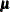, <cov=>)

Multivariate normal distribution with mean vectorand covariance matrixThe RANDOM statement syntax is illustrated as follows for one effect, where s2u can be a constant or a model parameter and zipcode is a data set variable that indicates group membership of the random effect u:

random u ~ normal(0,var=s2u) subject=zipcode;

The syntax is illustrated as follows for multiple effects, where mu and cov can be either parameters in the model or constant arrays:

array w;
array mu;
array cov[2,2];
random w ~ mvn(mu, cov) subject=zipcode;

Hyperparameters in the prior distribution of a random effect cannot be other random effects in the model. For example, the following statements are not allowed because the random effect g appears in the distribution for the random effect u:

random g ~ normal(0,var=s2g) subject=day;
random u ~ normal(g,var=s2u) subject=zipcode;

This restriction means that you cannot use multiple random statements to carry out an analysis that involves hierarchical centering. However, the hyperparameters can be model parameters (parameters that are declared in the PARMS statements). For a hierarchical centering example that involves multiple-level random effects, see Nonlinear Poisson Regression Random-Effects Model.

The following options are available in the RANDOM statement:

INITIAL=SAS-data-set | constant | numeric-list

specifies the initial values of the random-effects parameters.

If you use a SAS data set, the data set must consist of variable names that agree with the random-effects parameters in the model (see the NAMESUFFIX= option for the naming convention of the random-effects parameters). You can provide a subset of the initial values.

For example, the following statement creates a data set with initial values for the random-effects parameters u_1, u_2, and u_3:

data RandomInit;
input u_1 u_2 u_3;
datalines;
2.3 3 -3
;

The following RANDOM statement takes the values in the RandomInit data set to be the initial values of the corresponding random-effects parameters in the model:

random u ~ normal(0,var=s2u) subject=index init=randominit;

Specifying a constant assigns that constant as the initial value to all random-effects parameters in the statement. For example, the following statement assigns the value 5 to be used as an initial value for all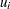in the model:

random u ~ normal(0,var=s2u) subject=index init=5;

If you have multiple effects, you can provide a list of numbers that have the same length as the dimension of your random-effects array. Each number is then given to all corresponding random-effects parameters in order. For example, the following statement assigns the value 2 to be used as an initial value for all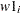and the value 3 to be used for all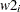in the model:

array w w1 w2;
random w ~ mvn(mu, cov) subject=index init=(2 3);
MONITOR= (symbol-list)

outputs analysis for selected random-effects parameters. You can choose either to monitor all random-effects parameters by specifying monitor=(u), where u is the random-effect symbol or array, or to monitor a subset of the parameters by specifying a variable list. The following statement outputs analysis for parameters u_1, u_2, u_3, and u_23:

random u ~ normal(0,var=s2u) subject=index  monitor=(u_1-u_3 u_23);

The naming convention in the symbol-list must agree with the NAMESUFFIX= option, which controls how the parameter names of the random-effect are created. By default, NAMESUFFIX=SUBJECT, and the symbol-list must use suffixes that correspond to values in the SUBJECT= data set variable. With the NAMESUFFIX=POSITION option, the symbol-list must use suffixes that agree with the input order of the SUBJECT= variable. If the SUBJECT= variable has a character value, you cannot use the hyphen (-) in the symbol-list to indicate a range of variables.

By default, PROC MCMC does not monitor any random-effects parameters. When used, this option takes the specification of the STATISTICS= and PLOTS= options in the PROC MCMC statement.

PROC MCMC outputs all the posterior samples of random-effects parameters to the OUTPOST= output data set.

NAMESUFFIX=value

specifies how the names of the random-effects parameters are internally created. PROC MCMC creates the names by concatenating the random-effect symbol with an underscore and a series of numbers. The following values control the type of numbers that are used in such contruction:

SUBJECT

constructs the parameter names by appending the values of the SUBJECT= variable in the input data set.

POSITION

constructs the parameter names by appending the numbers 1, 2, 3, and so on, where the number indicates the order in which the SUBJECT= variable appears in the data set.

For example, suppose that you have an input data set with four observations, and the SUBJECT= variable zipcode takes on four values: 27513, 27515, 27513, and 27514. The following SAS statement creates three random-effects parameters named u_27513, u_27515, and u_27514:

random u ~ normal(0,var=s2u) subject=zipcode namesuffix=subject;

On the other hand, using NAMESUFFIX=POSITION creates three parameters named as u_1, u_2, and u_3.

By default, NAMESUFFIX=SUBJECT.

SUBJECT=effect

identifies the subjects in the random-effects model. The random-effects parameters associated with each subject are assumed to be conditionally independent of each other given other parameters in the model (parameters that are defined by the PARMS statement). The SUBJECT= variable can be either a numeric variable or character literal, and it does not need to be sorted.